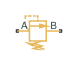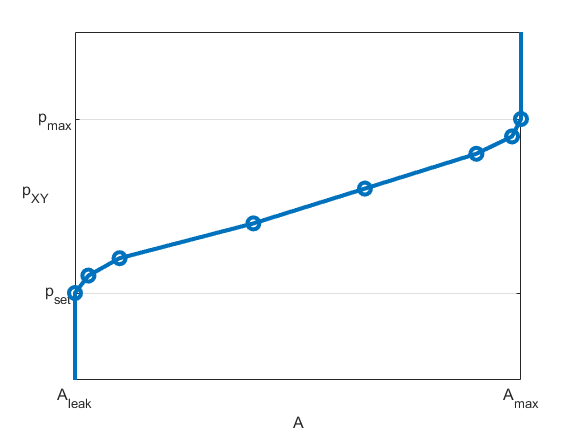# Pressure Relief Valve (TL)

Pressure relief valve in a thermal liquid network

•Libraries:
Simscape / Fluids / Thermal Liquid / Valves & Orifices / Pressure Control Valves

## Description

The Pressure Relief Valve (TL) block represents a valve that relieves excess pressure in a thermal liquid network. The valve remains closed when the pressure is less than a specified value. When this pressure is met or surpassed, the valve opens. This set pressure is either a threshold pressure differential over the valve, between ports A and B, or between port A and atmospheric pressure. A control pressure above the set pressure causes the valve to gradually open, which allows the fluid network to relieve excess pressure.

### Mass Balance

The mass conservation equation in the valve is

`${\stackrel{˙}{m}}_{A}+{\stackrel{˙}{m}}_{B}=0,$`

where:

• ${\stackrel{˙}{m}}_{A}$ is the mass flow rate into the valve through port A.

• ${\stackrel{˙}{m}}_{B}$ is the mass flow rate into the valve through port B.

### Mass Flow Rate

The block calculates the mass flow rate as

`$\stackrel{˙}{m}=\frac{{C}_{d}{A}_{valve}\sqrt{2\overline{\rho }}}{\sqrt{P{R}_{loss}\left(1-{\left(\frac{{A}_{valve}}{{A}_{port}}\right)}^{2}\right)}}\frac{\Delta p}{{\left[\Delta {p}^{2}+\Delta {p}_{crit}^{2}\right]}^{1/4}},$`

where:

• Cd is the value of the Discharge coefficient parameter.

• Avalve is the instantaneous valve open area.

• Aport is the value of the Cross-sectional area at ports A and B parameter.

• $\overline{\rho }$ is the average fluid density.

• Δp is the valve pressure difference pApB.

The critical pressure difference, Δpcrit, is the pressure differential associated with the Critical Reynolds number, Recrit, the flow regime transition point between laminar and turbulent flow:

`$\Delta {p}_{crit}=\frac{\pi }{8{A}_{valve}\overline{\rho }}{\left(\frac{\mu {\mathrm{Re}}_{crit}}{{C}_{d}}\right)}^{2},$`

where μ is the dynamic viscosity of the thermal liquid.

The pressure loss, PRloss, describes the reduction of pressure in the valve due to a decrease in area. The block calculates PRloss as

`$P{R}_{loss}=\frac{\sqrt{1-{\left(\frac{{A}_{valve}}{{A}_{port}}\right)}^{2}\left(1-{C}_{d}^{2}\right)}-{C}_{d}\frac{{A}_{valve}}{{A}_{port}}}{\sqrt{1-{\left(\frac{{A}_{valve}}{{A}_{port}}\right)}^{2}\left(1-{C}_{d}^{2}\right)}+{C}_{d}\frac{{A}_{valve}}{{A}_{port}}}.$`

The pressure recovery describes the positive pressure change in the valve due to an increase in area. When you clear the Pressure recovery check box, the block sets PRloss to 1.

### Opening Parameterization

When you set Opening parameterization to ```Linear - Area vs. pressure```, the block calculates the opening area as

`${A}_{valve}=\stackrel{^}{p}\left({A}_{\mathrm{max}}-{A}_{leak}\right)+{A}_{leak},$`

where Aleak is the value of the Leakage Area parameter and Amax is the value of the Maximum opening area parameter. The normalized pressure, $\stackrel{^}{p}$, is

`$\stackrel{^}{p}=\frac{{p}_{control}-{p}_{set}}{{p}_{Max}-{p}_{set}},$`

where the expressions for pcontrol, pset, and pMax depend whether the setting of the Pressure control specification parameter is `Pressure at port A` or ```Pressure differential```.

pcontrol is the valve control pressure:

where pAtm is the atmospheric pressure.

pset is the set pressure:

pMax is the maximum pressure:

This figure shows how the block controls the opening area using the linear parameterization.When the valve is in a near-open or near-closed position in the linear parameterization, you can maintain numerical robustness in your simulation by adjusting the parameter. If the parameter is nonzero, the block smoothly saturates the control pressure between pset and pmax. For more information, see Numerical Smoothing.

When you set Opening parameterization to `Tabulated data - Area vs. pressure`, the block calculates the opening area as

`${A}_{valve}=tablelookup\left({p}_{control,TLU,ref},{A}_{TLU},{p}_{control},interpolation=linear,extrapolation=nearest\right),$`

where:

• pcontrol,TLU,ref = pTLU + poffset.

• pTLU is the Pressure differential vector parameter.

• poffset is an internal pressure offset that causes the valve to start closing when pcontrol,TLU,ref = pset.

• ATLU is the Opening area vector parameter.

This figure shows how the block controls the opening area when Opening parameterization is ```Tabulated data - Area vs. pressure```.When you set Opening parameterization to `Tabulated data - Volumetric flow rate vs. pressure`, the valve opens according to the user-provided tabulated data of volumetric flow rate and pressure differential between ports A and B.

The block calculates the mass flow rate as

`$\stackrel{˙}{m}=\overline{\rho }\stackrel{˙}{V},$`

where:

• $\stackrel{˙}{V}$ is the volumetric flow rate.

• $\overline{\rho }$ is the average fluid density.

The block calculates the relationship between the mass flow and pressure using

`$\stackrel{˙}{m}=K\overline{\rho }\sqrt{\Delta p},$`

where

`$\text{K=}\frac{\stackrel{˙}{V}}{\sqrt{|\Delta p|}}.$`

### Opening Dynamics

When you set Opening dynamics to `On`, the block introduces a control pressure lag where pcontrol becomes the dynamic control pressure, pdyn. The instantaneous change in dynamic opening area is calculated based on the Opening time constant parameter, τ:

`${\stackrel{˙}{p}}_{dyn}=\frac{{p}_{control}-{p}_{dyn}}{\tau }.$`

By default, the block clears the Opening dynamics check box.

### Energy Balance

The energy conservation equation in the valve is

`${\varphi }_{A}+{\varphi }_{B}=0,$`

where:

• ϕA is the energy flow rate into the valve through port A.

• ϕB is the energy flow rate into the valve through port B.

### Predefined Parameterization

You can populate the block with pre-parameterized manufacturing data, which allows you to model a specific supplier component.

1. In the block dialog box, next to Selected part, click the "<click to select>" hyperlink next to Selected part in the block dialogue box settings.

2. The Block Parameterization Manager window opens. Select a part from the menu and click Apply all. You can narrow the choices using the Manufacturer drop down menu.

3. You can close the Block Parameterization Manager menu. The block now has the parameterization that you specified.

4. You can compare current parameter settings with a specific supplier component in the Block Parameterization Manager window by selecting a part and viewing the data in the Compare selected part with block section.

Note

Predefined block parameterizations use available data sources to supply parameter values. The block substitutes engineering judgement and simplifying assumptions for missing data. As a result, expect some deviation between simulated and actual physical behavior. To ensure accuracy, validate the simulated behavior against experimental data and refine your component models as necessary.

## Ports

### Conserving

expand all

Thermal liquid conserving port associated with valve inlet A.

Thermal liquid conserving port associated with valve inlet B.

## Parameters

expand all

Method of modeling the valve opening or closing. You can parameterize the valve opening linearly or by using a table of values that correlate the area-to-pressure differential.

Specification method for the valve control pressure. When you set this parameter to `Pressure at port A`, the block uses only the pressure at port A to determine when the flow exceeds the value of the Set pressure (gauge) parameter. When you set this parameter ```Pressure differential```, the block uses the value of the Set pressure differential parameter to determine whether the pressure differential from port A to port B exceeds the range.

#### Dependencies

To enable this parameter, set Opening parameterization to ```Linear - Area vs. pressure``` or ```Tabulated data - Area vs. pressure```.

Pressure at which the opening mechanism triggers. The opening area remains variable throughout the pressure regulation range of the valve.

#### Dependencies

To enable this parameter, set either:

• Pressure control specification to `Pressure at port A` and Opening parameterization to ```Linear - Area vs. pressure```

• Set Opening parameterization to ```Tabulated data - Volumetric flow rate vs. pressure```

Minimum pressure differential between ports A and B required to open the valve. A pressure differential above this value causes the valve to gradually open until it reaches the fully open state.

#### Dependencies

To enable this parameter, set Pressure control specification to ```Pressure differential``` and Opening parameterization to ```Linear - Area vs. pressure```.

Pressure interval over which the valve opening area varies. The interval begins at the value of the Set pressure (gauge) parameter or when the difference between ports A and B exceeds the value of the Set pressure differential parameter.

#### Dependencies

To enable this parameter, set Opening parameterization to ```Linear - Area vs. pressure```.

Opening area of the valve in the fully open position due to sealing imperfections, when the valve is at the upper limit of the pressure regulation range.

#### Dependencies

To enable this parameter, set Opening parameterization to ```Linear - Area vs. pressure```.

Sum of all gaps when the valve is in the fully shut position. The block saturates smaller numbers to this value. This parameter contributes to numerical stability by maintaining continuity in the flow.

#### Dependencies

To enable this parameter, set Opening parameterization to ```Linear - Area vs. pressure```.

Vector of pressure differential values for the tabular parameterization of the valve opening area. The vector elements must correspond one-to-one with the elements in the Opening area vector parameter. The elements must be listed in ascending order and must be greater than 0. The block employs linear interpolation between table data points.

#### Dependencies

To enable this parameter, set either:

• Opening parameterization to ```Tabulated data - Volumetric flow rate vs. pressure```

• Opening parameterization to ```Tabulated data - Area vs. pressure``` and Opening pressure specification to ```Pressure differential```

Vector of pressure differential values for the tabular area parameterization of the valve opening area. The vector elements must correspond one-to-one with the elements in the Opening area vector parameter. The elements are must be in ascending order and must be greater than 0. The block employs linear interpolation between table data points.

#### Dependencies

To enable this parameter, set Opening parameterization to ```Tabulated data - Area vs. pressure```, and Opening pressure specification to ```Pressure at port A```.

Vector of valve opening areas for the tabular area parameterization of the valve opening area. The vector elements must correspond one-to-one with the elements in the Pressure differential vector parameter. The elements must be in ascending order and must be greater than 0. The block employs linear interpolation between table data points.

#### Dependencies

To enable this parameter, set Opening parameterization to ```Tabulated data - Area vs. pressure```.

Vector of volumetric flow rate values for the tabular parameterization of the valve opening. This vector must have the same number of elements as the Pressure drop vector parameter. The vector elements must be in ascending order.

#### Dependencies

To enable this parameter, set Opening parameterization to ```Tabulated data - Volumetric flow rate vs. pressure```.

Area at ports A and B, which the block uses in the pressure-flow rate equation that determines the mass flow rate through the valve.

Correction factor that the block uses to account for discharge losses in theoretical flows.

#### Dependencies

To enable this parameter, set Opening parameterization to ```Linear - Area vs. pressure``` or ```Tabulated data - Area vs. pressure```.

Upper Reynolds number limit for laminar flow through the valve.

#### Dependencies

To enable this parameter, set Opening parameterization to ```Linear - Area vs. pressure``` or ```Tabulated data - Area vs. pressure```.

Continuous smoothing factor that introduces a layer of gradual change to the flow response when the valve is in near-open or near-closed positions. Set this value to a nonzero value less than one to increase the stability of your simulation in these regimes.

A smoothing factor of 0 corresponds to a linear function that is discontinuous at the set and maximum-area pressures. A smoothing factor of 1 corresponds to a nonlinear function that changes continuously throughout the entire function domain.

A smoothing factor between 0 and 1 corresponds to a continuous function with smooth nonlinear transitions at the set and maximum-area pressures.

#### Dependencies

To enable this parameter, set Opening parameterization to ```Linear - Area vs. pressure```.

Whether to account for pressure increase when fluid flows from a region of smaller cross-sectional area to a region of larger cross-sectional area. To capture the increase in pressure, select this parameter.

#### Dependencies

To enable this parameter, set Opening parameterization to ```Linear - Area vs. pressure``` or ```Tabulated data - Area vs. pressure```.

Whether to account for transient effects to the fluid system due to the valve opening. Selecting this parameter approximates the opening conditions by introducing a first-order lag in the flow response.

Time constant by which to compute the lag in the opening dynamics.

#### Dependencies

To enable this parameter, select Opening dynamics.

## Version History

Introduced in R2016a

expand all